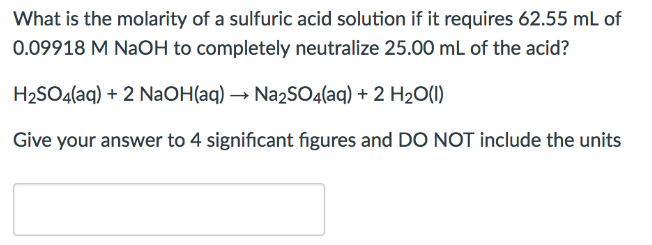# Problem: What is the molarity of a sulfuric acid solution if it requires 62.55 mL of 0.09918 M NaOH to completely neutralize 25.00 mL of the acid? H2SO4 (aq) + 2NaOH (aq) → Na2SO4 (aq) + 2H2O (l) Give your answer to 4 significant figures and DO NOT include the units.

###### FREE Expert Solution
95% (56 ratings)###### Problem Details

What is the molarity of a sulfuric acid solution if it requires 62.55 mL of 0.09918 M NaOH to completely neutralize 25.00 mL of the acid?

H2SO4 (aq) + 2NaOH (aq) → Na2SO4 (aq) + 2H2O (l)

Give your answer to 4 significant figures and DO NOT include the units.